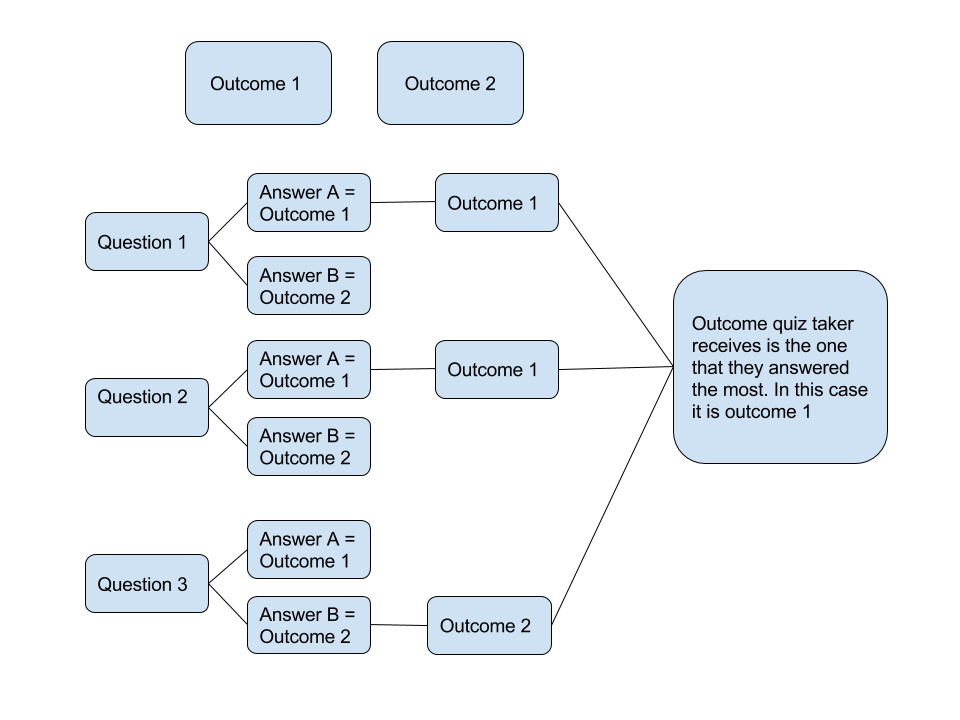# How Does The Quiz Logic Work?

### Learn how LeadQuizzes quiz logic works so you can create the best quiz!

Right now the quiz is set up so that it is results-based. Meaning each answer correlates with a result and the result with the most answers will be served to the quiz taker.

Below is a diagram showing how this works:What Happens In The Event Of A Tie?

If there is a tie, meaning the majority a quiz takers answers match with two or more outcomes the same number of times then the tie goes to the first question that resulted in that tie and the outcome associated with that question answer.

For example say you have a 5 question quiz and 3 potential outcomes. If a quiz taker answers to question 1 and it matches with outcome 1 and questions 2 through 5 are an equal combination of outcomes 2 and 3. Then the tie goes to the outcome that question 2 is mapped with since question 1 was not a question that resulted in the tie.